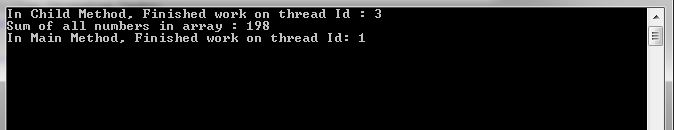Task in C# Extended is the continuation of my previous article on Tasks, where we discussed about the basic understanding of Tasks and its usage. Continuing with that, here we will discuss some more functionality and behavior of Task.

### How to wait for Tasks to complete ?

As you know that Task runs asynchronously on a thread from the Thread Pool . The thread starts task execution asynchronously along with the main application thread as soon as it has been instantiated and invoked.

We also see an example in our previous discussion on Task, where both threads (Main and Child) started its execution simultaneously (asynchronously), the Main thread didn’t wait for the Child thread to complete. The Child thread continued its execution even after completion of Main thread execution.

In cases, where you want your main application thread execution to wait until other child Tasks completes its execution, then you can do that in Task by using Wait() method.

### Example – Waiting for Task to complete :

In below example, we have created a method as PrintOddEvenNumbers that prints odd and even numbers. In the Main method, we have created a task instance Task t = new Task(PrintOddEvenNumbers); and then invoking it by calling t.start()  .

In the above example, we have used t.Wait() method for Task t object. Which will make the Main thread to wait until the Child thread finishes its execution.

When we run the above program, we will get below output.If you notice in the above output console, here the the Main thread waits for the Child thread to finish its execution.

### Get return value from Tasks ?

We can also return a value from a Task object, In that case, we have to specify the return type as a type parameter to the Task object as Task<T> , where T  being the type.

### Example : Return value from a Task :

In below example, we have created a method as SumOfAllNumber that takes an integer array as input and calculates the sum of values present in the array, finally returns the calculated sum value. In the Main method, we have created a task instance Task<int> task = new Task<int>(() => SumOfAllNumber(arrNum)); . If you can see, here we have declared as Task<int> which means the return type we are expecting is of type int .

When we run above example then will get below output.In the above console window, we can see that both Main method and Task executed on different thread. However since we are using Task.Result property in the Main method to read the return value from the Task. So, that’s why it blocked the Main method until it finished execution.